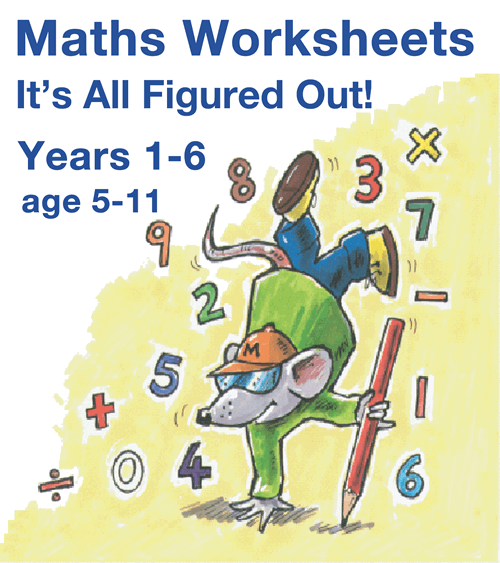# It's All Figured Out!

### Maths Worksheets for Years 1-6 ages 5-11

Great news: up-to-date and available at URBrainy.com

A superb set of maths resources, containing over 5 000 photocopiable worksheets. Whether you are looking for inspiration for your class teaching, homework sheets, differentiated maths for groups in your classroom, or help for special needs or high flyers, then this is the resource for you. Ideal for parents to support their children at home.

### Updated and now available at URBrainy.com

All these resources and more now available online at URBrainy.com

## Free URBrainy Trial

Free trial available, but at £6 for a month they are tremendous value and allow access to all other year groups, plus English as well.

## The very best primary maths worksheets

The new curriculum is here and we have updated all six year groups to ensure that the challenges ahead can be met confidently and successfully. Our worksheets help children become proficient in all areas of Maths including geometry and statistics.

• Updated for the very latest statutory requirements with many new pages added
• Answers included to make marking simple
• Concept pages clearly explain the ideas being taught
• Makes differentiation in the classroom easy
• Reduces the teacher’s workload and saves valuable preparation time
• MathSphere has been a favourite resource for teachers for over 15 years## Includes All Our Worksheets

With this product you get access to all our worksheets for years 1 to 6. You can find out more about each year group's worksheets by visiting their product page.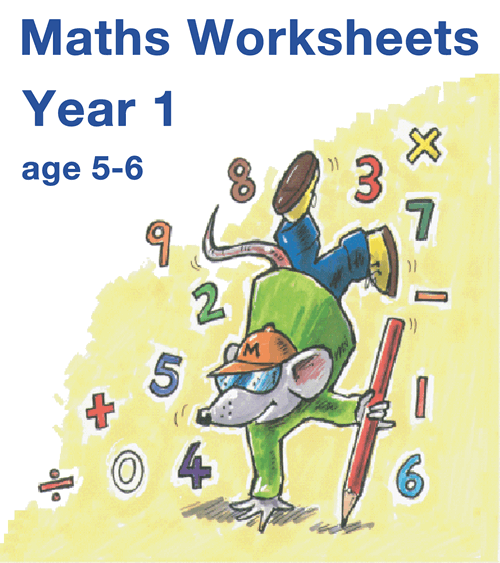#### Year 1 Maths Worksheets

A superb set of worksheets for maths in year 1 (aged 5‐6)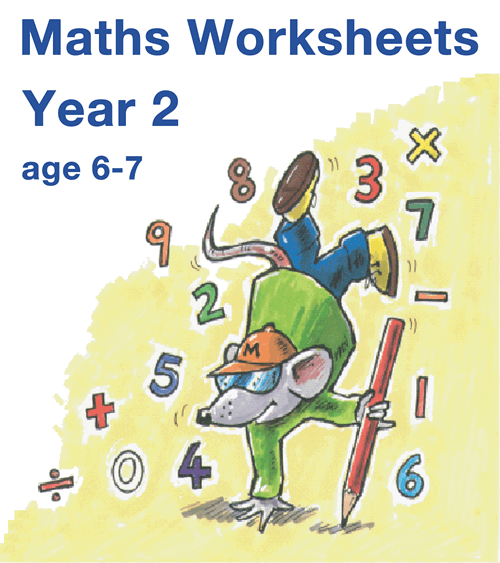#### Year 2 Maths Worksheets

Over 800 pages of resources for year 2 (aged 6‐7) children.#### Year 3 Maths Worksheets

Meets the new primary curriculum, with over 1000 pages.#### Year 4 Maths Worksheets

A vast collection of worksheets covering year 4 maths.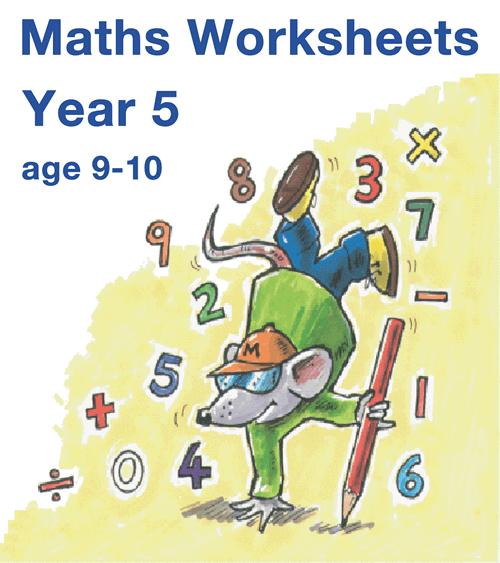#### Year 5 Maths Worksheets

The 2014 Programme of Study is fully covered.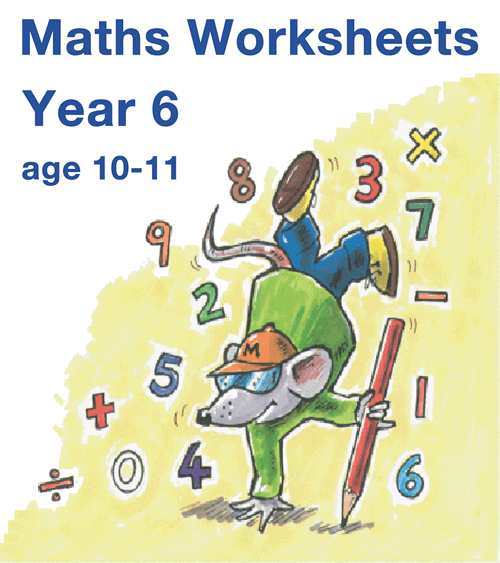#### Year 6 Maths Worksheets

A real challenge for year 6 children (aged 10‐11).

## Try Some Free Sample Worksheets

To give you a better idea of the worksheets available we have provided a selection of free modules for Years 1 to 6.

Please download these samples, print them out and use at home, or photocopy and use in the classroom.24 Pages

### Year 1

#### Number Sequences

Using number lines to hop along in twos, threes and fives as well as extending number sequences.23 Pages

### Year 2

#### Solve Money Problems

Beginning by using coins to help count before moving onto pounds and pence and the decimal point.6 Pages

### Year 3

#### 8 × Tables To 12

Plenty of help with learning the 8 times table up to 12 × 8.18 Pages

### Year 4

#### Use Known Facts To Add & Subtract

Practice with calculating rapidly using a range of methods.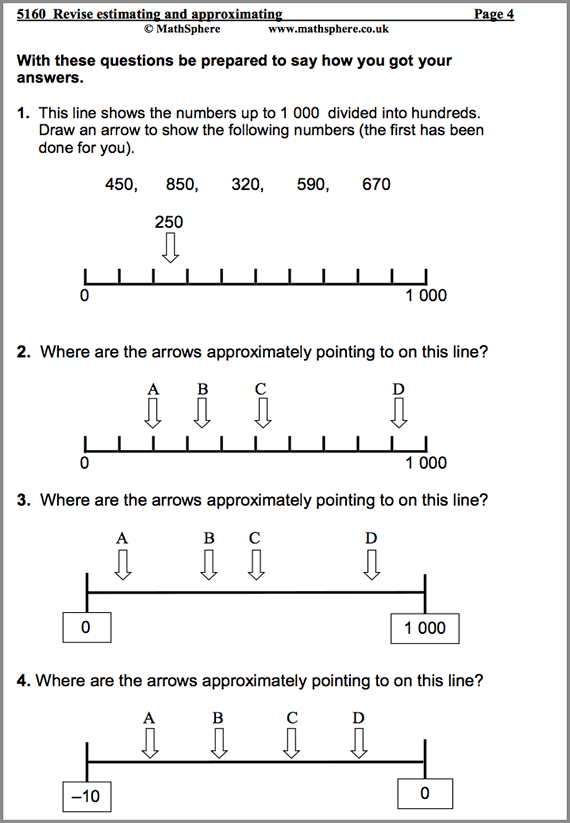11 Pages

### Year 5

#### Revise Estimating & Approximating

Estimating on number lines and plenty of practical ideas to challenge children.28 Pages

### Year 6

#### Rounding Decimals

Understanding the relationship between decimals and fractions is crucial for future success.

## List of modules

It is impossible to describe in a single page the massive amount of high quality maths contained in this one product as it remains one of the most popular resources in UK schools.

To give you some idea, have a look at the list of all modules here.

### Number and place value 265 pages

 Count reliably a set of objects. Count in steps of one. 16 Read and write numbers in figures and words. 22 Counting over ten. 22 Comparing and ordering numbers. More than, less than. 20 Counting in twos. 23 One or ten more than, or less than a given number. 16 Counting in tens; odd and even numbers. 14 Counting in fives. 6 Count back in ones, twos and tens. 15 Order a set of familiar numbers. 16 Describe and extend number sequences. 24 Estimation and approximation. 13 Know the number names and recite them. 17 Nursery rhymes with number and counting. 22 Solve mathematical puzzles and investigate. 19

### Multiplication and division 56 pages

 Doubles and halves. 22 Combining groups of 2, 5 and 10. 16 Using arrays. 7 Understanding division; sharing into equal groups. 11

### Measures 91 pages

 Measure and compare. 9 Suggest suitable units to estimate and measure. 13 Use simple measuring equpiment. 10 Understand and read the time. 18 Word problems involving measurement. 20 Problems involving money. 21

### Mental arithmetic 72 pages

 Autumn term. 24 Spring term. 24 Summer term. 24

### Addition and subtraction 181 pages

 Understand addition. 12 Understand subtraction. 11 Know addition and subtraction facts. 24 Add or subtract 9. 20 Add or subtract pairs of numbers mentally. 23 Understand that more than two numbers can be added together. 12 Use knowledge that addition can be done in any order. 8 Adding or subtracting 10. 14 Adding or subtracting crossing the tens. 15 Add or subtract a multiple of 10. 13 Choose and use appropriate number operations to solve problems. 8 Investigate general statements. 10 Solve simple word problems. 11

### Fractions 25 pages

 Recognise simple fractions and equivalence. 15 Recognise and find simple fractions. Count in halves. 10

### Geometry 40 pages

 Describe 3D and 2D shapes. 16 Make models and describe the features of shapes. 11 Describe position and direction. 7 Describe movement and turning. 6

### Using and Applying Mathematics 29 pages

 Length, number and ordering word searches. 8 Investigate 3 digits. 5 Investigate totals to 20. 5 Caterpillar investigation. 5 Penny sweets investigation. 6

### Statistics 23 pages

 Collect and sort information (1) 12 Collect and sort information (2) 11

### Number and Place Value 166 pages

 Revise counting on and back in steps of one. 18 Counting in tens and twos. Odd and even numbers. 16 Counting in steps of three and five. 9 Describe and extend number sequences. Count on and back. 19 Read and write 2-digit numbers. Partition numbers. 22 Comparing and ordering numbers. 19 One or ten more than or less than. Position numbers. 22 Order a set of familiar numbers. 15 Estimation and approximation. 15

### Multiplication and Division 129 pages

 Derive doubles and halves quickly. 12 Revise understanding multiplication. 17 2 times table up to 12. 9 5 times table up to 12. 9 10 times table up to 12 9 Tables: 2x, 5x and 10x speed sheets. 13 Understand division. 25 Division tables 5 Multiply and divide mentally. 16

### Measurement 108 pages

 Measure and compare. 15 Suggest suitable units to estimate and measure. 10 Use measuring equipment . Interpret scales. 14 Understand and read the time. 12 More understanding and reading the time 16 Word problems involving measurement. 13 Solve problems involving money. 23

### Statistics 29 pages

 Collect, sort and organise information. 9 Represent data. 13

### Mental arithmetic 75 pages

 Autumn term 25 Spring term 25

### Fractions 32 pages

 Recognise simple fractions and equivalence. 14 Recognise thirds and halves. 7 Count in quarters. 6

### Geometry 58 pages

 Describe and classify 2D and 3D shapes. 10 Make shapes and patterns. Describe features. 11 Describe movement in a straight line and angle as a measure of turn 11 Describe position and direction. 11 Symmetry 11

### Using and Applying Maths 93 pages

 Choose and use appropriate number operations to solve problems. 15 Investigate totals 10 Investigate: 5 digit addition. 4 Investigate consecutive numbers. 4 Solve mathematical puzzles and investigate. 21 Investigate general statements. 12 Solve simple word problems. 14 Maths vocabulary word searches. 8

### Number and Place Value 156 pages

 Revise counting on and back. 18 Read and write 3-digit numbers. Partition into HTU. 15 Counting in tens and hundreds. Odd and even numbers. 19 Count in fours and eights. 7 Count in fifties. 6 Describe and extend number sequences. 14 Comparing and ordering numbers. 16 One, ten or one hundred more than or less than. 18 Order a set of familiar numbers. 9 Estimation and approximation. 11 Rounding a number to the nearest 10 and 100. 13 Further rounding. 10

### Multiplication and Division 173 pages

 Revise understanding of multiplication. 16 Know multiplication facts by heart. Relate to division. 8 3x and 4x tables up to 12. 13 8x table up to 12. 6 Tables: speed sheets. 21 Revise division. 15 Strategies for multiplication and division. 16 Multiply and divide by 10 and 100. Doubling and halving. 21 Understand remainders. Rounding after division. 15 Develop written methods for multiplication 6 Written methods for multiplication. 9 Written division using repeated subtraction. 6 Simple written division. 5 Formal method of short division. 6 Choose appropriate number operations. 10

### Measurement 133 pages

 Measure and compare. 17 Choose and use suitable units to estimate and measure. 7 Use measuring equipment . Interpret scales. 10 Solve problems involving money. 20 Measuring perimeter. 8 Solve problems involving measurement. 13 Understand and read the time. 16 Read the time from clocks, calendars and timetables. 26 Time: a.m. and p.m. and 24 hour clock. 9 Roman numerals and time. 7

### Statistics 33 pages

 Collect, sort and organise data. 10 Use bar charts, tally charts, bar graphs and pictograms. 15 Use Venn and Carroll diagrams. 8

### Mental arithmetic 75 pages

 Autumn term. 25 Spring term. 25 Summer term. 25

### Fractions 47 pages

 Recognise simple fractions and equivalence. 13 Recognise and find simple fractions. 12 Find unit fractions of numbers or quantities. 10 Adding fractions. 4 Subtracting fractions. 4 Ordering fractions. 4

### Geometry 67 pages

 Describe and classify 3D and 2D shapes. 12 Make shapes and describe their features. 12 Describe movement and understand angle as a measure of turn. 9 Describe position and direction. 9 Perpendicular and parallel lines. 7 Symmetry. 11 Pentominoes. 7

### Using and Applying Mathematics 104 pages

 Solve mathematical puzzles and investigate. 23 Investigate general statements. 10 Investigate addition. 11 Investigate subtraction. 10 Investigate multiplication. 4 Solve word problems. 16 Maths vocabulary word searches. 8 Investigate column addition. 4 Routes investigation. 5 Investigate coins. 4 More coins. 5 Add one to four. 4

### Number and Place Value 94 pages

 Counting in multiples of 7, 8, 9 and 25. 10 Find 1, 10, 100 or 1000 more. 16 Read and write whole numbers. Partition. 14 Revise vocabulary for comparing and ordering numbers. 13 Recognise and order negative numbers. 13 Extend number sequences. 12 Revise rounding numbers. 11 Roman Numerals. 5

### Multiplication and Division 188 pages

 7x table. 12 9x table. 14 11x and 12x tables up to 12. 13 Tables: speed sheets. 21 More multiplication facts. Doubling and halving. 11 Multiply and divide mentally. 13 Multiply and divide by 10 and 100 and 1000. 16 Develop written methods of multiplication. 9 Short multiplication 2-digits by 1-digit. 8 Short multiplication 3-digits by 1-digit. 9 Revise understanding of division. 12 Understand remainders. 19 Develop written methods of division. 8 Formal method of short division with no remainders. 7 Formal method of short division with remainders. 6 Odd and even. Multiples and tests of divisibility. 10

### Measurement 87 pages

 Standard metric units of measurement. 11 Units to estimate and measure. 8 Suggest suitable measuring equipment and record results. 20 Measure and calculate the perimeter and area of simple shapes. 16 Measurement investigation. 4 Solve problems involving length, mass or capacity. 7 Using the 24-hour clock. 14 Solve problems involving time. 7

### Statistics 21 pages

 Collect, sort and organise data. Use tables, graphs and charts (1) 11 Collect, sort and organise data. Use tables, graphs and charts (2) 10

### Mental arithmetic 75 pages

 Autumn term. 25 Spring term. 25 Summer term. 25

### Addition and Subtraction 140 pages

 Revise addition and its relationship to subtraction. 15 Revise subtraction and its relationship to addition. 12 Add or subtract the nearest multiple of 10 and adjust. 13 Use the relationship between addition and subtraction. 21 Use knowledge of addition and subtraction and place value. 19 Use known number facts to add and subtract mentally. 18 Refine and use efficient written methods of addition 13 Formal written addition of 4-digit numbers. 7 Refine and use efficient written methods of subtraction. 12 Formal written subtraction of 4-digit numbers. 10

### Fractions 101 pages

 Revise equivalent fractions. 12 Count in hundredths. 4 Find fractions of numbers or quantities. 7 Identify pairs of fractions that total 1. 10 Add and subtract fractions. 12 Use decimal notation. Order decimal fractions. 12 Decimal fractions. 12 Recognise equivalence between decimals and fractions. 13 Divide by 10 and 100. 7 Solve problems involving money. 8 Round decimals. 4

### Geometry 78 pages

 Describe and classify 3D and 2D shapes. 16 Visualise 3-D objects and make nets of common solids. 10 Make turns; estimate, draw and measure angles. 18 Symmetry; reflections and translations. 16 Recognise position and direction; use co-ordinates. 13 Triangle investigation. 5

### Using and Applying Mathematics 111 pages

 Choose appropriate number operations. 11 Choose method of calculating, including using calculators. 11 Explain methods and reasoning about numbers. 8 Investigate the multiplication square. 8 Solve problems, recognise patterns, generalise and predict. 10 Solve one and two step word problems. 8 Make and investigate general statements about numbers and shapes. 10 Revise checking results of calculations. 11 Develop calculator skills 14 Maths vocabulary games. 8 Lines and rectangles investigation. 4 Palindromic numbers. 8

### Number and Place Value 92 pages

 Read and write whole numbers. Partition. 21 Revise comparing and ordering numbers. 10 Count in powers of ten. 4 Extend number sequences. 12 Recognise and order negative numbers. 13 Solve 'real life' problems 8 Rounding numbers. 7 Revise estimating and approximating. 11 Roman Numerals. 6

### Multiplication and Division 176 pages

 Revise understanding multiplication. 13 Revise understanding division. 12 Revise multiplying and dividing by 10 and 100. 12 More multiplying and dividing by 10 and 100. Double and halve. 13 Multiply and divide by 10, 100 and 1000. 9 Double and halve. Use factors to help calculate. 18 Use knowledge of tables to calculate mentally. 17 Square numbers, prime numbers, factors and multiples. 12 Revise and refine written methods of multiplication. 11 Short multiplication. 5 Written multiplication of decimals. 8 Long multiplication. 8 More long multiplication. 8 Revise written methods for division. 8 Revise short division. 7 Divide a 4-digit number by a 1-digit number. 4 Square and cube numbers, square roots and prime factors. 11

### Measurement 104 pages

 Units to measure length, mass or capacity. 20 Standard metric units of measurement. 10 Metric and Imperial. 5 Read scales, record, estimate and measure. 16 Perimeter and area. 18 Estimating and measuring time. 9 Solve problems involving time. 8 Solve problems involving length, mass or capacity. 7 Solve problems involving money. 8 Investigate cuboids. 3

### Statistics 46 pages

 Collect and organise data. Use tables, graphs and charts (1) 11 Further data handling. Use median and mode. 10 Interpreting graphs, tables and charts. (Also Booster material) 21 Two dice investigation. 4

### Fractions 83 pages

 Recognise equivalent fractions. Order fractions. 13 Find fractions of numbers or quantities. 6 Adding fractions. 7 Subtracting fractions. 7 Multiplying fractions. 5 Round decimals. Equivalence between decimals and fractions. 16 Order decimals: thousandths. 9 Understand percentages. 13 Find percentages of numbers or quantities. 7

### Geometry 61 pages

 Describe and classify 3D and 2D shapes. 11 Estimate, draw and measure angles. 10 Making shapes and patterns. 7 Recognise position and direction. Use co-ordinates. 13 Reflective symmetry. Reflections and translations. 15 Tessellation investigation. 5

### Using and Applying Mathematics 58 pages

 Checking results of calculations. 11 Use a calculator. 9 Probability: using the language of chance or likelihood. 7 Maths vocabulary games. 8 Investigate zero. 4 Investigate 10. 4 Investigate products. 3 Triangle numbers. 12

### Mental arithmetic 75 pages

 Autumn term. 25 Spring term. 25 Summer term. 25

### Number and Place Value 43 pages

 Read and write very large numbers. 5 Revise rounding whole numbers. 12 Recognise and order negative numbers. 17 Revise estimating and approximating. 9

### Fractions 149 pages

 Use fraction notation.Recognise equivalent fractions. 22 Find fractions of numbers or quantities. 9 Adding fractions. 8 Fraction investigation 1. 3 Investigate halves. 3 Subtracting fractions. 12 Multiplying and dividing fractions. 7 Use decimal notation. Order decimal fractions. 14 Use known facts to multiply and divide decimals. 14 Round decimals. Equivalence between decimals and fractions. 28 Understand remainders. Round up or down. 17 Percentages. 12

### Algebra 52 pages

 Make and investigate general statements. 13 Extend number sequences. 12 Algebra. 24 Algebra investigation. 3

### Geometry 86 pages

 Visualise, describe and classify 3D and 2D shapes. 8 More shapes and nets. 11 More measuring angle. 16 Investigate exterior and interior angles. 6 More properties of shapes. 16 Reflective symmetry. Reflections and translations. 16 Use co-ordinates and extend to four quadrants. 13

### Using and Applying Mathematics 105 pages

 Solve problems involving ‘real life’. 8 Solve problems involving money. 8 Solve problems involving time. 8 More time problems. 8 Choose appropriate methods of calculating. 11 Explain methods and reasoning. 7 Solve problems, generalise and predict. 11 Checking results of calculations. 11 Develop calculator skills. 9 Calculator fractions. 3 Maths vocabulary games. 8 Investigate factors and primes. 7 Investigate four fours. 3 Primes from squares. 3

### 209 pagesAddition, Subtraction, Multiplication and Division

 Revise addition. 16 Revise subtraction. 12 Use known number facts to add and subtract decimals. 11 Relationship between addition and subtraction. Add several numbers. 14 Revise multiplication. 13 Use known facts to multiply mentally. 19 Revise division. 12 Revise doubling and halving. Using factors. 14 Square numbers, prime numbers and identifying factors. 15 Use formal written methods of addition. 10 Use formal written methods of subtraction. 16 Use formal written methods of multiplication. 6 Long multiplication. 8 Use formal written methods of long division. 5 More long division: 3-digits divided by 2-digit numbers. 8 Division with decimals. 8 Multiples, factors and tests of divisibility. 12 Conventions for working out expressions. (Bodmas) 10

### Ratio and Proportion 53 pages

 Begin to understand ratio and proportion. 11 Solve problems concerning ratio and proportion. 9 Ratio and proportion problems. 12 Scale/Proportion/Conversion of units. 13 Scale drawing. 8

### Measurement 93 pages

 Use standard metric units and convert. 8 Units to estimate and measure length, mass and capacity. 8 Solve measurement problems. 7 Read scales, record estimates and measure. 14 Measure and calculate the perimeter and area of simple shapes. 15 More perimeter and area. 25 Measure and calculate the circumference of circles. 11 Farmer's field investigation. 5

### Statistics 37 pages

 Collect, sort and organise data. Pie charts. 11 Further data handling. 11 Probability. 9 Investigate measurement statistics. 6

### Mental arithmetic 75 pages

 Autumn term. 25 Spring term. 25 Summer term. 25

### Quick Details

• Over 5000 worksheets